# 7.1: Basic Properties of Vectors

•• Contributed by CK12
• CK12

An airplane being pushed off course by wind and a swimmer’s movement across a moving river are both examples of vectors in action.  Points in the coordinate plane describe location.  Vectors, on the other hand, have no location and indicate only direction and magnitude.  Vectors can describe the strength of forces like gravity or speed and direction of a ship at sea.  Vectors are extremely useful in modeling complex situations in the real world.

What are other differences between vectors and points?

### Properties of Vectors

A two dimensional vector is represented graphically as an arrow with a tail and a head.  The head is the arrow and is also called the terminal point.  When finding the vector between two points start with the terminal point and subtract the initial point (the tail).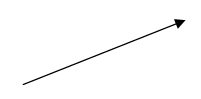The two defining characteristics of a vector are its magnitude and its direction.  The magnitude is shown graphically by the length of the arrow and the direction is indicated by the angle that the arrow is pointing.  Notice how the following vector is shown multiple times on the same coordinate plane.  This emphasizes that the location on the coordinate plane does not matter and is not unique.  Each representation of the vector has identical direction and magnitude.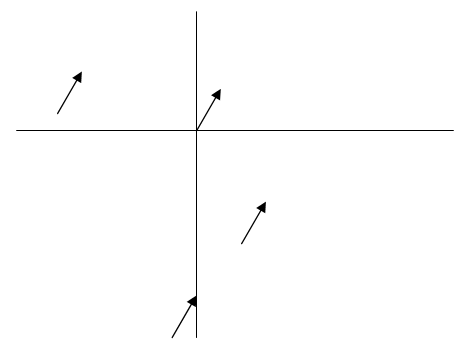One way to define a vector is as a line segment with a direction.  Vectors are said to be equal if they have the same magnitude and the same direction.  The absolute value of a vector is the same as the length of the line segment or the magnitude of the vector.  Magnitude can be found by using the Pythagorean Theorem or the distance formula.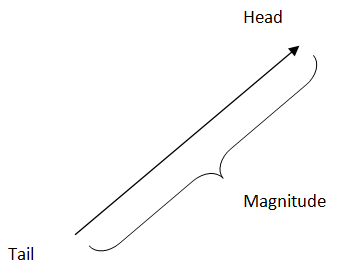There are a few different ways to write a vector $$v$$.

$$v, \vec{v}, \vec{v},$$ or $$v$$ with a $$\sim$$ underneath

When you write about vectors algebraically there are a few ways to describe a specific vector. First, you could describe its magnitude and angle as $$r, \theta$$. Second, you could describe it as an ordered pair: $$<x, y>$$. Notice that when discussing vectors you should use the brackets $$<>$$ instead of parentheses because it helps avoid confusion between a vector and a point. Vectors can be multidimensional.

Vectors are often used to describe the movement of objects. To describe the movement of a ship is traveling NNW at 17 knots (nautical $$\mathrm{mph}$$ ) as a vector, notice that NNW is halfway between $$\mathrm{NW}$$ and $$\mathrm{N}$$. When describing ships at sea, it is best to use bearing which has $$0^{\circ}$$ as due North and $$270^{\circ}$$ as due West. This makes NW equal to $$315^{\circ}$$ and NNW equal to $$337.5^{\circ}$$.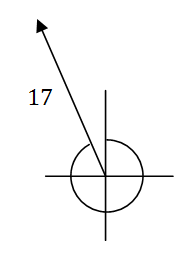When you see this picture, it turns into a basic trig question to find the $$x$$ and $$y$$ components of the vector. Note that the reference angle that the vector makes with the negative portion of the $$x$$ axis is $$67.5^{\circ}$$

$$\sin 67.5^{\circ}=\frac{y}{17}, \cos 67.5^{\circ}=\frac{x}{17}$$
$$<x, y>\approx<-6.5,15.7>$$

### Examples

Example 1

Earlier, you were asked what the differences between points and vectors are. There are many differences between points and vectors. Points are locations and vectors are made up of distance and angles. Parentheses are used for points and $$\langle>$$ are used for vectors. One relationship between vectors and points is that a point plus a vector will yield a new point. It is as if there is a starting place and then a vector tells you where to go from that point. Without the starting point, the vector could start from anywhere.

Example 2

Consider the points: $$A(1,3), B(-4,-6), C(5,-13)$$. Find the vectors in component form of $$\overrightarrow{A B}, \overrightarrow{B A}, \overrightarrow{A C}, \overrightarrow{C B}$$

Remember that when finding the vector between two points, start with the terminal point and subtract the initial point.

$$\overrightarrow{A B}=<-5,-9>$$
$$\overrightarrow{B A}=<5,9>$$
$$\overrightarrow{A C}=<4,-16>$$
$$\overrightarrow{C B}=<-9,7>$$

Example 3

A father is pulling his daughter up a hill. The hill has a $$20^{\circ}$$ incline. The daughter is on a sled which sits on the ground and has a rope that the father pulls as he walks. The rope makes a $$39^{\circ}$$ angle with the slope. A force diagram is a collection of vectors that each represent a force like gravity or wind acting on an object. Draw a force diagram showing how these forces act on the daughter's center of gravity:

a. The force of gravity.
b. The force holding the daughter in the sled to the ground.
c. The force pulling the daughter backwards down the slope.
d. The force of the father pulling the daughter up the slope.

The girl's center of gravity is represented by the black dot. The force of gravity is the black arrow straight down. The green arrow is gravity's effect pulling the girl down the slope. The red arrow is gravity's effect pulling the girl straight into the slope. The blue arrow represents the force that the father is exerting as he pulls the girl up the hill.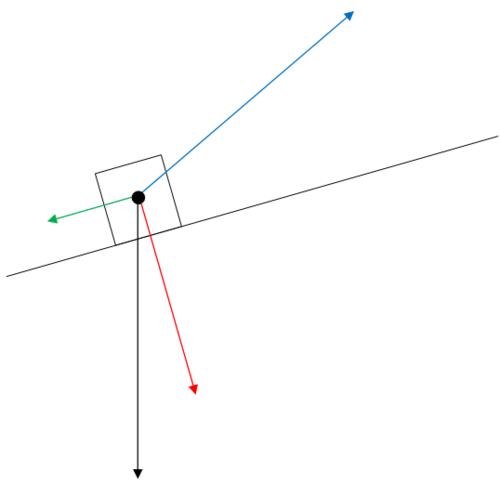Notice that the father’s force vector (blue) is longer than the force pulling the girl down the hill.  This means that over time they will make progress and ascend the hill.  Also note that the father is wasting some of his energy lifting rather than just pulling.  If he could pull at an angle directly opposing the force pulling the girl down the hill, then he would be using all of his energy efficiently.

Example 4

Center the force diagram from the previous question into the origin and identify the angle between each consecutive force vector.

The $$x$$ and $$y$$ axis are included as reference and note that the gravity vector overlaps with the negative $$y$$ axis. In order to find each angle, you must use your knowledge of supplementary, complementary and vertical angles and all the clues from the question. To check, see if all the angles sum to be $$360^{\circ}$$.

Example 5

Given the following vectors and point, compute the sum.

$$\begin{array}{l} A=(1,3), \vec{v}=<4,8>, \vec{u}=<-1,-5> \\ A+\vec{v}+\vec{u}=? \\ A=(1,3), \vec{v}=<4,8>, \vec{u}=<-1,-5>. A+\vec{v}+\vec{u}=(4,6) \end{array}$$

Review

1. Describe what a vector is and give a real-life example of something that a vector could model.

Consider the points: $$A(3,5), B(-2,-4), C(1,-12), D(-5,7)$$. Find the vectors in component form of:

2. $$\overrightarrow{A B}$$

3. $$\overrightarrow{B A}$$

4. $$\overrightarrow{A C}$$

5. $$\cdot \overrightarrow{C B}$$

6. $$\cdot \overrightarrow{A D}$$

7. $$\overrightarrow{D A}$$

8. What is $$C+\overline{C B}$$ ? Compute this algebraically and describe why the answer makes sense.

9. Use your answer to the previous problem to help you determine $$D+\overrightarrow{D A}$$ without doing any algebra.

10. A ship is traveling SSW at 13 knots. Describe this ship's movement in a vector.

11. A vector that describes a ships movement is $$<5 \sqrt{2}, 5 \sqrt{2}>$$. What direction is the ship traveling in and what is its speed in knots?

For each of the following vectors, draw the vector on a coordinate plane starting at the origin and find its magnitude.

12. $$<3,7>$$

13. $$<-3,4>$$

14. $$<-5,10>$$

15. $$<6,-8>$$